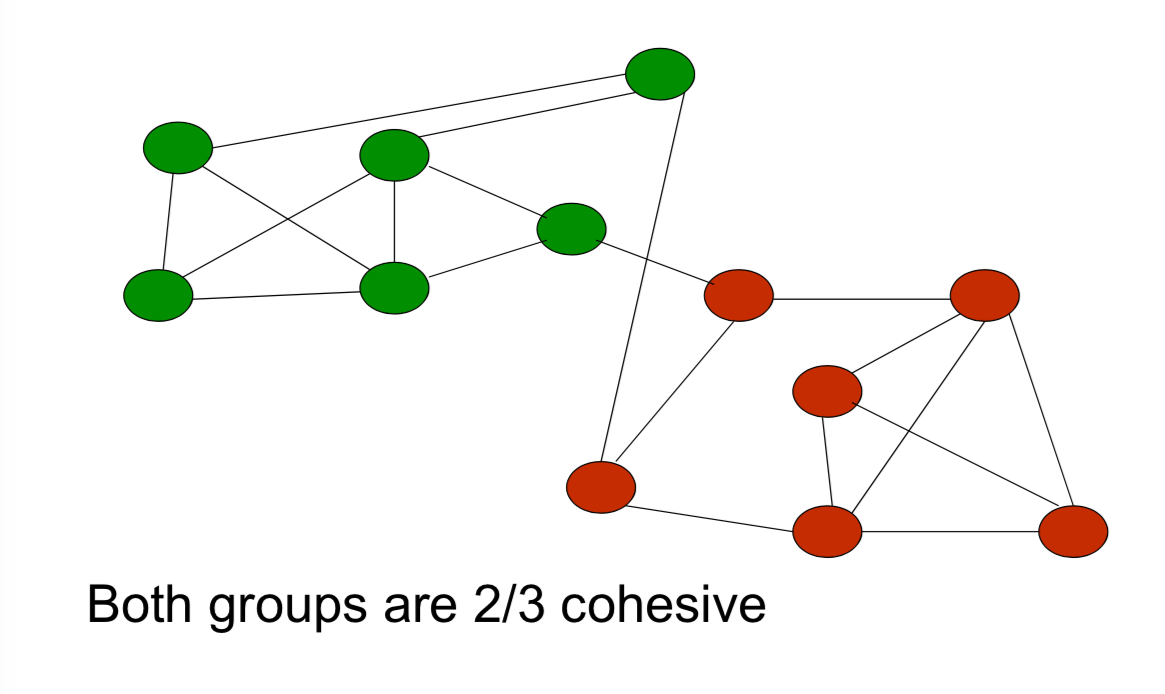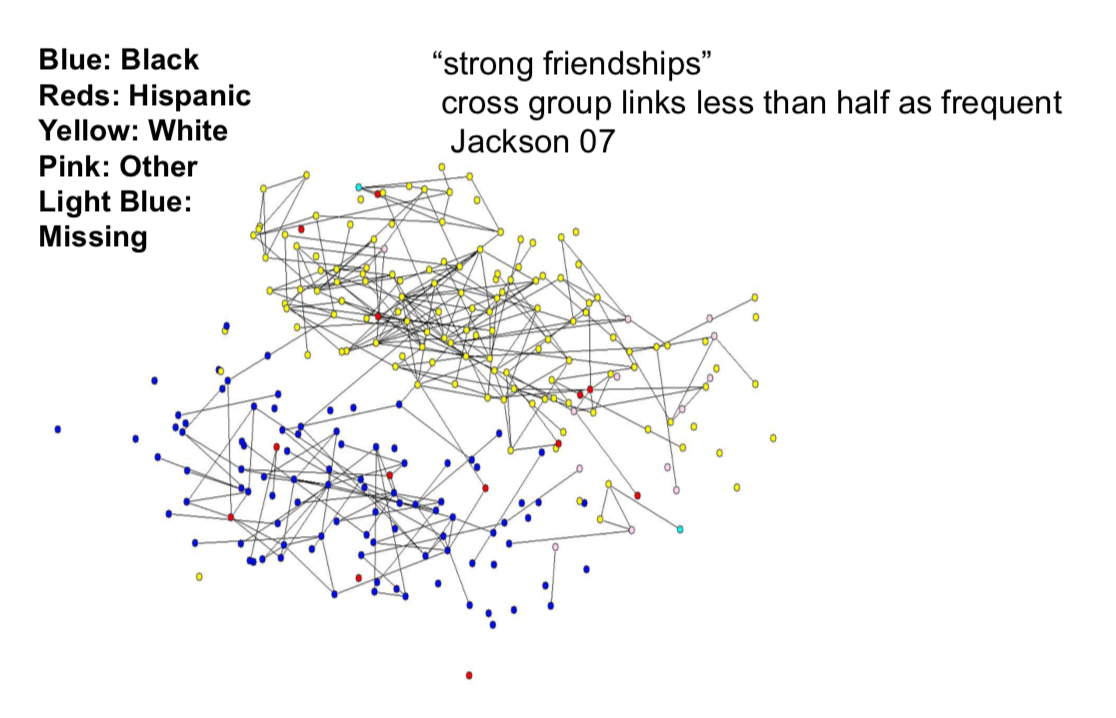# Games on Networks - Outline¶

• Basic Definitions

• Examples

• Strategic Complements / Substitutes

• Equilibrium existence and structure

• Equilibrium response to network structure

there's multiple different actions that can be sustained in a given network. So when is that it's possible that some people adopt a new technology and other people don't? Or that some people are, are becoming educated, other people are not, and so forth. So when is it that we actually can sustain multiple actions, even when we've got a lot of homogeneity in the society. Even when anybody has the same preferences and so forth, we still end up with different people taking different actions based on their position in the network, okay. So this is just sort of an interesting question conceptually to understand when this can happen.

# When can multiple actions be sustained¶

• Morris(2000) Coordination game

• Care only about fraction of neighbors

• prefer to take action 1 if fraction q or more take 1

• So if the majority of your friends take action one, you want to do that. If the majority of your friends take action zero, then you prefer to take action zero. So this is a game of strategic compliments. And a very simple one where everybody just cares about the fraction. So everybody's threshold is just a fraction of their degree. It's the same fraction.

And let me say a little bit about the background of this game. the game where it's actually a half is also what's known as the majority game. And this is a game which has been studied quite a bit in the statistical physics literature. And has some background in the, physics and, and, agent based, literatures. And, you know, part of the reason is that, that, there's certain kinds of particles. Where the particles might be sitting in some sort of lattice structure. And the particles react to what other particles are doing. So, if other particles end up in one state, then they end up trying to match the state or they could end up going in opposite directions, but in certain situations they'll flip into be in a certain state if, if more of their the other, so as more of their neighboring particles become excited, they become excited, for instance. And depending on what that threshold is, then that ends up having a percolation so that you can end up having this move through different kinds of of materials. And so that's been an area of study in physics. And this actually has a nice interesting relationship to these kinds of games on networks, where an, a given node cares about what its, its neighbors are doing, and would like to match actions to the neighbors.

# Equilibrium Structure¶

Let S be the group that take action 1

• Each i in S must have fraction of at least q neighbors in S

• Each i not in S must have a fraction of at least 1-q neighbors outside of S

# Cohesion¶

A group S is r-cohesive relative to g if

$min_{i.in.S}$ |{j in $N_i(g)$ and S}| / $d_i(g)$ $\ge$ r

At least a fraction r of each member of S's neighbors are in S

Cohesiveness of S is $min_{i.in.S}$ |j in $N_i(g)$ and S}| / $d_i(g)$# Equilibria where both strategies are played¶

Morris(2000): there exists a pure strategy equilibrium where both actions(0 and 1) are played if and only if there is a group S that is at least q cohesive and such that its complement is at least 1-q cohesive.

So it has to be that everybody in that set has at least q of their neighbors. So, this group S is going to be the group that plays action one. They want to play action one if and only if at least q of their neighbors do. So that set has to be q cohesive. Everybody has to have at least q of their neighbors in that set.
10:14 The compliment of S, has to be the people playing z, action zero. So none of them can have more than Q of their friends in, in the set. So that means that they have to have at least one minus Q of their friends outside. So this proposition just follows pretty much directly from the definition of the game and it's a very simple, straight forward calculation. But what it does, is it, it shows that this, now we've got a, a notion of cohesiveness of groups inside a network which is going to be very useful in identifying when you can sustain multiple equalibria in a, in a game.

# Homophily¶

• If q=1/2 - players want to match majority

• Then two groups that have more self-ties than cross-ties suffices to sustain both actions

• As q rises (game payoffs become more asymmetric), need more homophilous behavior between the groups to sustain both actions